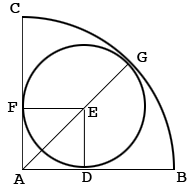SEARCH HOMEMath Central Quandaries & QueriesQuestion from Abhijeet: ABC is a quarter circle and a smaller circle is inscribed in it. if AB = 1cm then find the radius of smaller circleHi,

In my diagram $E$ is the center of the smaller circle. If the radius of the smaller circle the $|DE| = |EF| = |EG| = r.$ Angle $ADE$ is a right angle. By the symmetry the measure of the angle $EAD$ is $45^{o}.$Can you complete the problem now?

Penny* Registered trade mark of Imperial Oil Limited. Used under license.Math Central is supported by the University of Regina and the Imperial Oil Foundation.